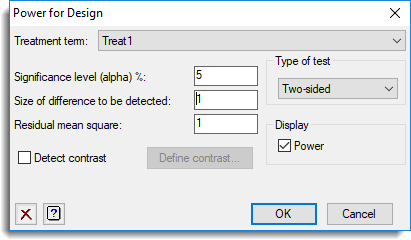1. Home
2. Power for Design

# Power for Design

This calculates the power for a given design, i.e. for a given level of response between two treatment groups and confidence level, it will calculate the probability of detecting this response. The design used is that specified in the Generate a Standard Design dialog.## Treatment term

A dropdown list of the treatment terms in the current design. The calculated power will be for a difference between two means in this treatment means table.

## Significance level (alpha)%

Significance level as a percentage (0-100) at which the response is required to be detected. The default is 5%.

## Size of difference to be detected

Size of the difference between treatment effects that is to be detected.

## Residual mean square

This the residual mean square expected for the stratum that contains the selected treatment term (evaluated from previous experience). If you have similar studies, these can be estimated from an analysis of variance. You can display the stratum variances from an analysis of variance to see the required values, or alternatively you can examine the analysis of variance table.

## Detect contrast

Calculate the power of a specified contrast between means in the treatment terms. Click Define contrast to open a menu where you can define a contrast variate.

## Type of test

Specifies the type of test. You can use a One-sided or Two-sided t-test. Alternatively, if you select F-ratio the size of difference is taken to be the maximum difference between the effects of treatment term, and uses an F-test. The other settings enable you to test for Equivalence or Non-inferiority. With equivalence, the size of difference provides a threshold below which the treatments can be assumed to be equivalent. For example, if the treatments have effects e1 and e2, the null hypothesis that the treatments are not equivalent is that either

(e1 – e2) ≤ – size of difference
or
(e1 – e2) ≥ – size of difference

with the alternative hypothesis that they are equivalent, i.e.

-size of difference < (e1 – e2) < size of difference

With non-inferiority, the size of difference again specifies the threshold for the effect of one treatment to be superior to another. For example, to demonstrate non-inferiority of treatment 1 compared to treatment 2, the null hypothesis becomes

(e1 – e2) ≥ -size of difference

which represents a simple one-sided t-test.

## Display

Specifies which items of output are to be displayed in the Output window.

 Power Display the probability of detection (power)